# MP Board Class 7th Maths Solutions Chapter 2 Fractions and Decimals Ex 2.5

## MP Board Class 7th Maths Solutions Chapter 2 Fractions and Decimals Ex 2.5

Question 1.
Which is greater?
(i) 0.5 or 0.05
(ii) 0.7 or 0.5
(iii) 7 or 0.7
(iv) 1.37 or 1.49
(v) 2.03 or 2.30
(vi) 0.8 or 0.88.
Solution:
(i) 0.5 or 0.05
Converting these decimal numbers into like fractions, we obtain(ii) 0.7 or 0.5
Converting these decimal numbers into like fractions, we obtain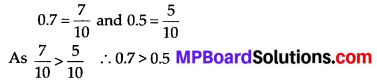(iii) 7 or 0.7
Converting these decimal numbers into like fractions, we obtain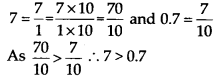(iv) 1.37 or 1.49
Converting these decimal numbers into like fractions, we obtain(v) 2.03 or 2.30
Converting these decimal numbers into like fractions, we obtain(vi) 0.8 or 0.88
Converting these decimal numbers into like fractions, we obtain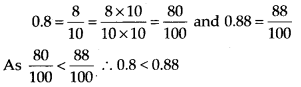Question 2.
Express as rupees using decimals:
(i) 7 paise
(ii) 7 rupees 7 paise
(iii) 77 rupees 77 paise
(iv) 50 paise
(v) 235 paise.
Solution:
There are 100 paise in 1 rupee. Therefore, if we want to convert paise into rupees, we have to divide paise by 100.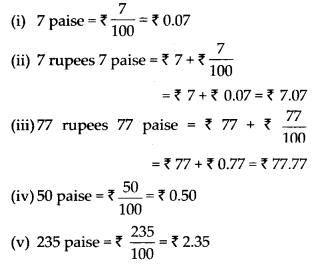Question 3.
(i) Express 5 cm in metre and kilometre.
(ii) Express 35 mm in cm, m and km.
Solution:Question 4.
Express in kg:
(i) 200 g
(ii) 3470 g
(iii) 4 kg 8g
Solution:Question 5.
Write the following decimal numbers in the expanded form:
(i) 20.03
(ii) 2.03
(iii) 200.03
(iv) 2.034
Solution: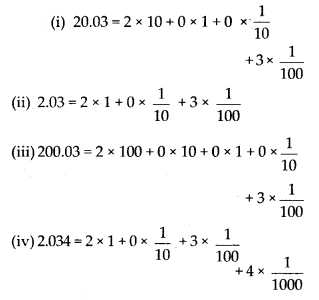Question 6.
Write the place value of 2 in the following decimal numbers:
(i) 2.56
(ii) 21.37
(iii) 10.25
(iv) 9.42
(v) 63.352.
Solution: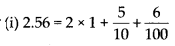∴ Place value of 2 in 2.56 is ones.∴ Place value of 2 in 21.37 is tens.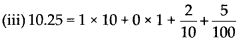∴ Place value of 2 in 10.25 is tenths.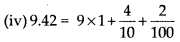∴ Place value of 2 in 9.42 is hundredths.∴ Place value of 2 in 63.352 is thousandths.Question 7.
Dinesh went from place A to place Band from there to place C. A is 7.5 km from B and B is
12.7 km from C. Ayub went from place A to place D and from there to place C. D is 9.3 km from A and C is 11.8 km from D. Who travelled more and by how much?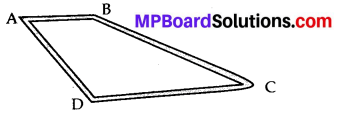Solution:
Distance travelled by Dinesh = AB + BC = (7.5 + 12.7) km = 20.2 km
Distance travelled by Ayub = AD + DC
= (9.3+ 11.8) km = 21.1 km
Difference = (21.1 – 20.2) km = 0.9 km
Hence, Ayub travelled 0.9 km more distance than Dinesh.Question 8.
Shyama bought 5 kg 300 g apples and 3 kg 250 g mangoes. Sarala bought 4 kg 800 g oranges and 4 kg 150 g bananas. Who bought more fruits?
Solution:
Total fruits bought by Shyama = 5 kg 300 g + 3 kg 250 g = 8 kg 550 gHence, Sarala bought more fruits.

Question 9.
How much less is 28 km than 42.6 km?
Solution:
(42.6 – 28.0) km = 14.6 km
Hence, 28 km is less than 42.6 km by 14.6 km.